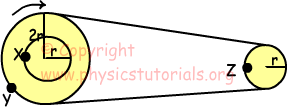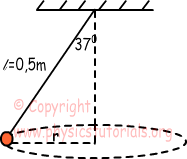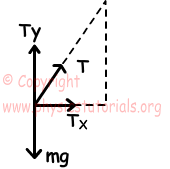## Rotational Motion Exam1 and Problem Solutions

Rotational Motion Exam1 and Problem Solutions

1. An object, attached to a 0,5m string, does 4 rotation in one second.Find

a) Period

b) Tangential velocity

c) Angular velocity of the object.

a) If the object does 4 rotation in one second, its frequency becomes;

f=4s-1 T=1/f=1/4s

b) Tangential velocity of the object;

V=2.π.f.r V=2.3.4.0,5 V=12m/s

c) Angular velocity of the object

2. Find the relation between tangential and angular velocities of points X, Y and Z.X and Y rotate together, so if X does one rotation then Y also does one rotation. On the contrary, if Y does one revolution, Z does two revolutions.

Angular velocities of the X, Y and Z are; ωX=ωY=ωZ/2

3. An object hanged on a rope L=0,5m, does rotational motion. If the angle between rope and vertical is 370, find the tangential velocity of the object. (g=10m/s2, cos370=0,8, sin370=0,6)Free body diagram of system is given below;Horizontal component of tension on the rope makes object rotate.

TX=mV2/r, TY=m.g

Radius of the motion path is;

r=L.sin370=0,5.0,6=0,3m

tan370=TX/TY

3/4=mV2/r/m.g

3/4=V2/g.r

V=3/2m/s

4. An object having mass m does rotational motion. Its angular velocity is ω and radius of motion path is r. Find kinetic energy of the object in terms of r, ω, and m.

EK=1/2m.V2

V=ω.r

EK=1/2m(ω.r)2

EK=mω2.r2/2

5. Stone having mass 0,5kg rotates in horizontal. It is hanged on 1m rope. If the tension on the rope is 80 N, find the frequency of the motion.

Fnet=80N=m.ω2.r

80=m.4.π2.f2.r

80=0,5.4.32.f2.1

f=2s-1

Author: